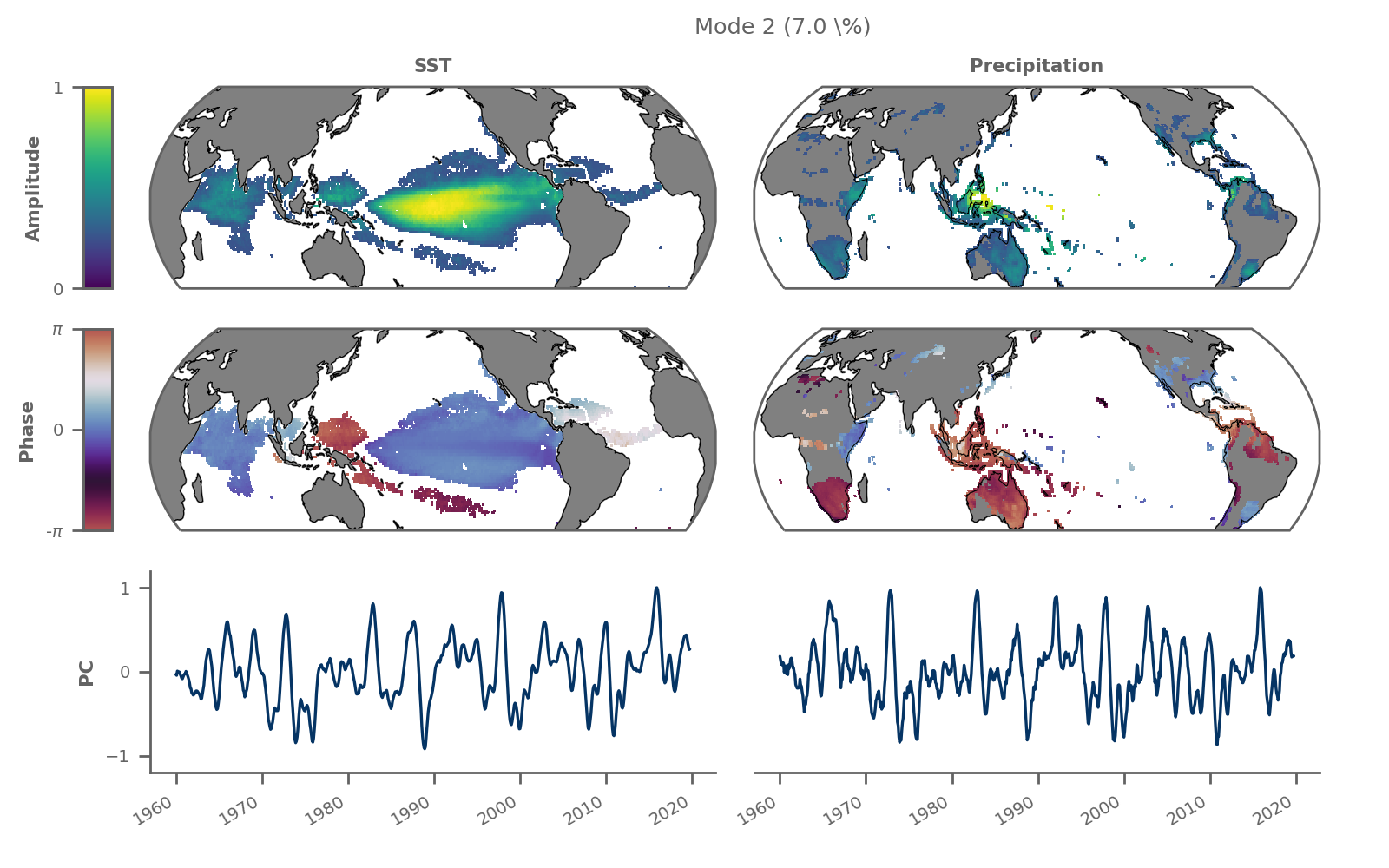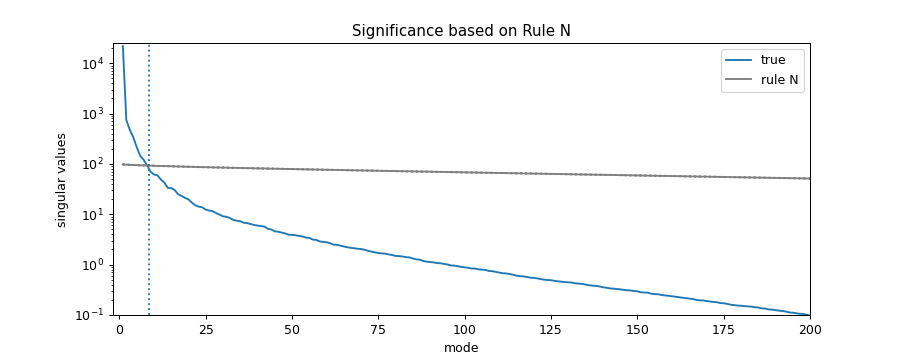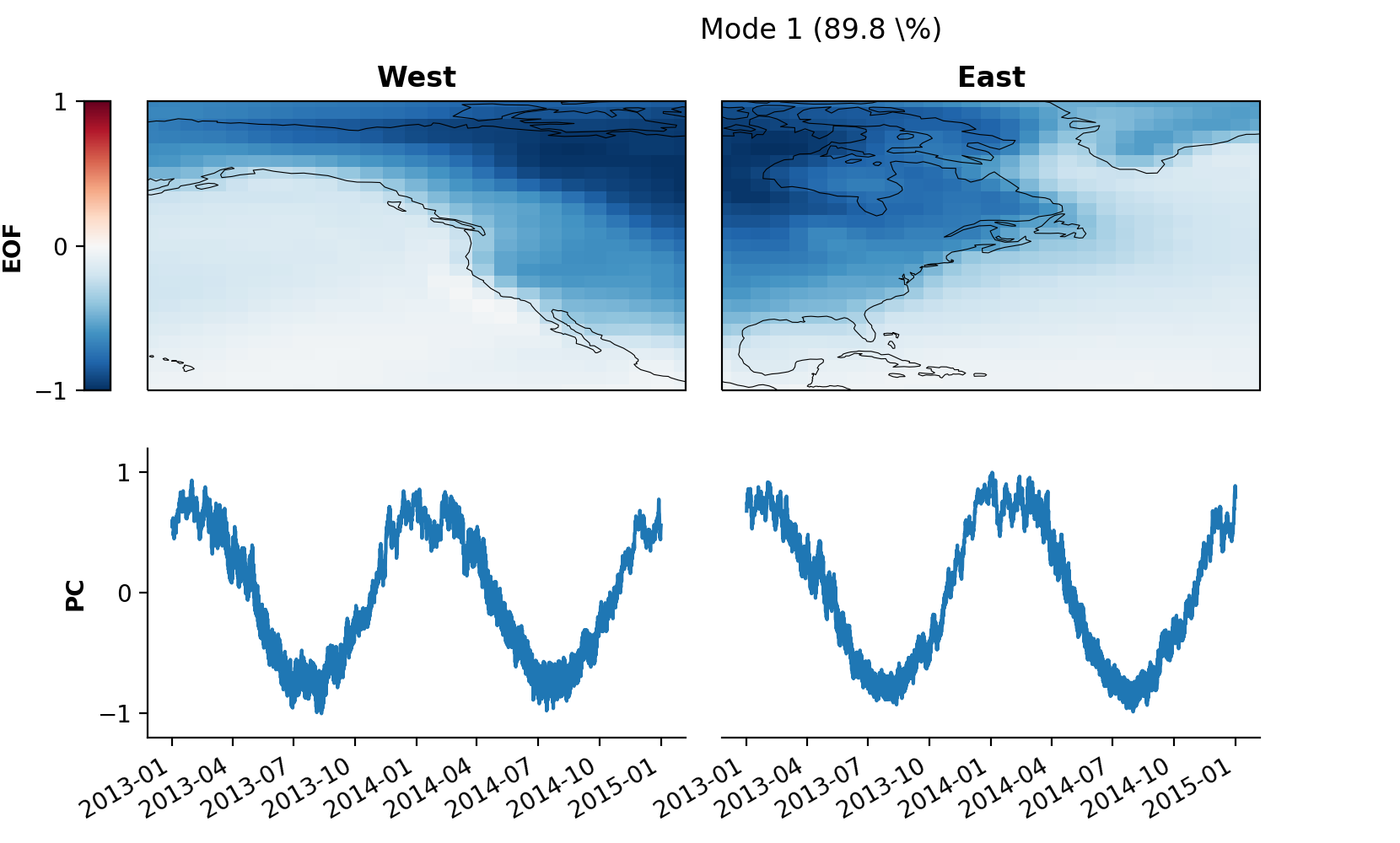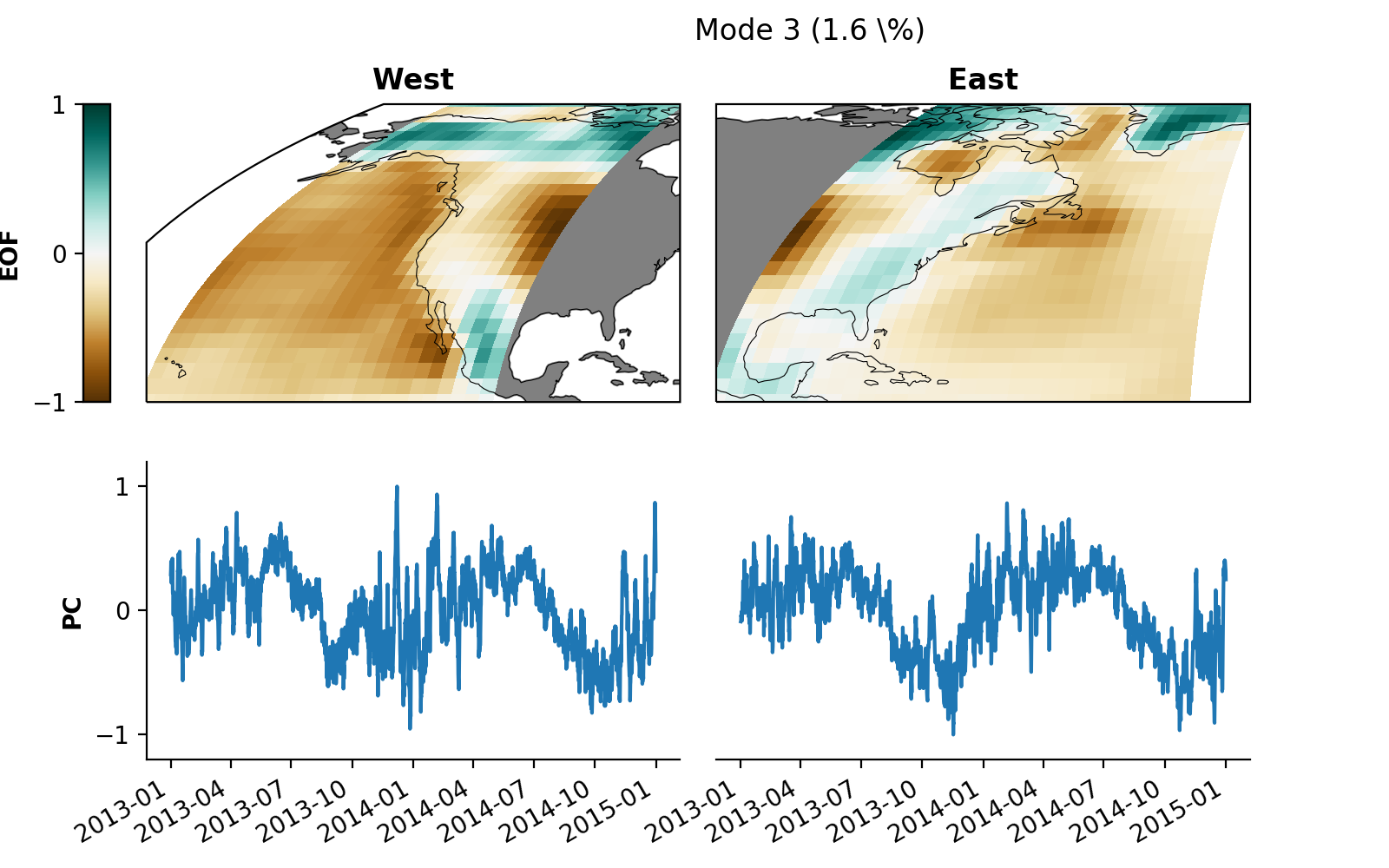# Xmca

Maximum Covariance Analysis in Python
Alternatives To Xmca
Quaternion5401737a month ago186April 28, 20223mitPython
Add built-in support for quaternions to numpy
Alyn174
4 years ago15mitPython
Detect and fix skew in images containing text
Quaternionic6122 months ago39March 27, 20224mitPython
Interpret numpy arrays as quaternionic arrays with numba acceleration
Eigen3topython38
4 months ago4bsd-2-clausePython
Provide Eigen3 to numpy conversion
Pymesh28
36 years ago7October 16, 20153mitPython
Library for manipulating (Translate, Rotate and Scale) 3D data using numpy. Currently, this library supports STL & OBJ.
Xmca20
a year ago6mitPython
Maximum Covariance Analysis in Python
Rowan11
4 months ago1otherPython
A Python package for working with quaternions.
Models10
3 years agoR
NLP models
Rotnist8
5 years agomitPython
Rotated MNIST dataset
Infogan Tensorflow5
4 years agoPython
InfoGAN implementation using tensorflow
Alternatives To Xmca
Select To Compare

Alternative Project Comparisons

# xMCA | Maximum Covariance Analysis in PythonThe aim of this package is to provide a flexible tool for the climate science community to perform Maximum Covariance Analysis (MCA) in a simple and consistent way. Given the huge popularity of `xarray` in the climate science community, `xmca` supports `xarray.DataArray` as well as `numpy.ndarray` as input formats.Mode 2 of complex rotated Maximum Covariance Analysis showing the shared dynamics of SST and continental precipitation associated to ENSO between 1980 and 2020.

## 🔰 What is MCA?

MCA maximises the temporal covariance between two different data fields and is closely related to Principal Component Analysis (PCA) / Empirical Orthogonal Function analysis (EOF analysis). While EOF analysis maximises the variance within a single data field, MCA allows to extract the dominant co-varying patterns between two different data fields. When the two input fields are the same, MCA reduces to standard EOF analysis.

For the mathematical understanding please have a look at e.g. Bretherton et al. or the lecture material written by C. Bretherton.

## ⭐️ New in release 1.4.x

• Much faster and more memory-efficient algorithm
• Significance testing of individual modes via
• Period parameter of `solve` method provides more flexibility to exponential extension, making complex MCA more stable
• Fixed missing coslat weighting when saving a model (Issue 25)

## 📌 Core Features

Standard Rotated Complex Complex Rotated
EOF analysis ✔️ ✔️ ✔️ ✔️
MCA ✔️ ✔️ ✔️ ✔️

* click on check marks for reference
** Complex rotated MCA is also available as a pre-print on arXiv.

## 🔧 Installation

Installation is simply done via

``````pip install xmca
``````

If you have problems during the installation please consult the documentation or raise an issue here on Github.

## 📰 Documentation

A tutorial to get you started as well as the full API can be found in the documentation.

## ⚡️ Quickstart

Import the package

``````    from xmca.array import MCA  # use with np.ndarray
from xmca.xarray import xMCA  # use with xr.DataArray
``````

As an example, we take North American surface temperatures shipped with `xarray`. Note: only works with `xr.DataArray`, not `xr.Dataset`.

``````    import xarray as xr  # only needed to obtain test data

# split data arbitrarily into west and east coast
data = xr.tutorial.open_dataset('air_temperature').air
west = data.sel(lon=slice(200, 260))
east = data.sel(lon=slice(260, 360))
``````

#### PCA / EOF analysis

Construct a model with only one field and solve it to perform standard PCA / EOF analysis.

``````    pca = xMCA(west)                        # PCA of west coast
pca.solve(complexify=False)            # True for complex PCA

svals = pca.singular_values()     # singular vales = eigenvalues for PCA
expvar      = pca.explained_variance()  # explained variance
pcs         = pca.pcs()                 # Principal component scores (PCs)
eofs        = pca.eofs()                # spatial patterns (EOFs)
``````

Obtaining a Varimax/Promax-rotated solution can be achieved by rotating the model choosing the number of EOFs to be rotated (`n_rot`) as well as the Promax parameter (`power`). Here, `power=1` equals a Varimax-rotated solution.

``````    pca.rotate(n_rot=10, power=1)

expvar_rot  = pca.explained_variance()  # explained variance
pcs_rot     = pca.pcs()                 # Principal component scores (PCs)
eofs_rot    = pca.eofs()                # spatial patterns (EOFs)
``````

#### MCA

Same as for PCA / EOF analysis, but with two input fields instead of one.

``````    mca = xMCA(west, east)                  # MCA of field A and B
mca.solve(complexify=False)            # True for complex MCA

eigenvalues = mca.singular_values()     # singular vales
pcs = mca.pcs()                         # expansion coefficient (PCs)
eofs = mca.eofs()                       # spatial patterns (EOFs)
``````

#### Significance analysis

A simple way of estimating the significance of the obtained modes is by running Monte Carlo simulations based on uncorrelated Gaussian white noise known as Rule N (Overland and Preisendorfer 1982). Here we create 200 of such synthetic data sets and compare the synthetic with the real singular spectrum to assess significance.

``````    surr = mca.rule_n(200)
median = surr.median('run')
q99 = surr.quantile(.99, dim='run')
q01 = surr.quantile(.01, dim='run')

cutoff = np.sum((svals - q99 > 0)).values  # first 8 modes significant

fig = plt.figure(figsize=(10, 4))
svals.plot(ax=ax, yscale='log', label='true')
median.plot(ax=ax, yscale='log', color='.5', label='rule N')
q99.plot(ax=ax, yscale='log', color='.5', ls=':')
q01.plot(ax=ax, yscale='log', color='.5', ls=':')
ax.axvline(cutoff + 0.5, ls=':')
ax.set_xlim(-2, 200)
ax.set_ylim(1e-1, 2.5e4)
ax.set_title('Significance based on Rule N')
ax.legend()
``````The first 8 modes are significant according to rule N using 200 synthetic runs.

``````    mca.save_analysis('my_analysis')    # this will save the data and a respective
# info file. The files will be stored in a
# special directory
mca2 = xMCA()                       # create a new, empty instance
# loaded via specifying the path to the
# info file created earlier
``````

#### Quickly inspect your results visually

The package provides a method to plot individual modes.

``````    mca2.set_field_names('West', 'East')
pkwargs = {'orientation' : 'vertical'}
mca2.plot(mode=1, **pkwargs)
``````Result of default plot method after performing MCA on T2m of North American west and east coast showing mode 1.

You may want to modify the plot for some better optics:

``````    from cartopy.crs import EqualEarth  # for different map projections

# map projections for "left" and "right" field
projections = {
'left': EqualEarth(),
'right': EqualEarth()
}

pkwargs = {
"figsize"     : (8, 5),
"orientation" : 'vertical',
'cmap_eof'    : 'BrBG',  # colormap amplitude
"projection"  : projections,
}
mca2.plot(mode=3, **pkwargs)
``````You can save the plot to your local disk as a `.png` file via

``````    skwargs={'dpi':200}
mca2.save_plot(mode=3, plot_kwargs=pkwargs, save_kwargs=skwargs)
``````

I am just starting my career as a scientist. Feedback on my scientific work is therefore important to me in order to assess which of my work advances the scientific community. As such, if you use the package for your own research and find it helpful, I would appreciate feedback here on Github, via email, or as a citation:

Niclas Rieger, 2021: nicrie/xmca: version x.y.z. doi:10.5281/zenodo.4749830.

## 💪 Credits

Kudos to the developers and contributors of the following Github projects which I initially used myself and used as an inspiration:

And of course credits to the developers of the extremely useful packages

Popular Numpy Projects
Popular Rotation Projects
Popular Mathematics Categories
Related Searches

Get A Weekly Email With Trending Projects For These Categories
No Spam. Unsubscribe easily at any time.
Python
Numpy
Rotation
Pca